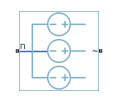# Voltage Source (Three-Phase)

Ideal three-phase voltage source with optional harmonics

• Library:
• Simscape / Electrical / Sources

•## Description

The Voltage Source (Three-Phase) block models an ideal three-phase voltage source or a three-phase voltage source with harmonics. You specify the configuration using the Source representation parameter.

When you select `None` for the Source Impedance parameter, the Voltage Source block models an ideal three-phase voltage source that maintains sinusoidal voltage of the specified magnitude across its terminals, independently of the current flowing through the source.

The source has a wye configuration, and port n provides a connection to the center of the wye. Port ~ is an expandable three-phase port representing the three phases, a, b, and c. The current is positive if it flows from the center of the wye to the positive terminal, and the voltage across each phase is equal to the difference between the voltage at the positive terminal and the center of the wye, V(+) – Vn.

### Equations

The output voltage for the Voltage Source block is defined by these equations:

`$v={v}_{DC}+{v}_{AC}\mathrm{sin}\left(2\pi ft+\varphi \right)+{v}_{N}$`
`${v}_{a}={V}_{0}\mathrm{sin}\left(2\pi ft+\phi \right)$`
`${v}_{N}=\sqrt{{P}_{v}/2}\frac{N\left(0,1\right)}{\sqrt{h}}$`
`${v}_{c}={V}_{0}\mathrm{sin}\left(2\pi ft+\phi +{120}^{\circ }\right),$`

where:

• V0 is the peak phase voltage.

• vline_rms is the root-mean square (RMS) phase-to-phase voltage.

• va, vb, vc are the respective phase voltages.

• f is frequency.

• φ is the phase shift.

• t is time.

When you specify the three-phase voltage source with harmonics representation, the output voltage for the Voltage Source block is defined by these equations:

`${V}_{0}=\frac{\sqrt{2}}{\sqrt{3}}{v}_{line_rms}{H}_{ratios}$`

`${v}_{a}={V}_{0}\mathrm{sin}\left(\left(2\pi ft+\phi \right){H}_{orders}^{\text{'}}\right)$`

`${v}_{b}={V}_{0}\mathrm{sin}\left(\left(2\pi ft+\phi -\theta \right){H}_{orders}^{\text{'}}\text{​}\right)$`

`${v}_{c}={V}_{0}\mathrm{sin}\left(\left(2\pi ft+\phi +\theta \right){H}_{orders}^{\text{'}}\right),$`

where:

• V0 is a row-vector containing the peak voltage of the fundamental and harmonic sinusoids.

• vline_rms is the RMS phase-to-phase voltage.

• Hratios is a row-vector of harmonic ratios. The first element is 1 to represent the fundamental.

• Horders is a row-vector of harmonic orders. The first element is 1 to represent the fundamental.

• va, vb, vc are the respective phase voltages.

• f is a column-vector of harmonic frequencies. The first element is the fundamental frequency.

• φ is a column-vector of harmonic phase shifts. The first element is the fundamental phase shift.

• θ is a column-vector of harmonic phase offsets. The first element is `120°`.

• t is the time.

When you select `X/R ratio` for the Source Impedance parameter, the equations for source impedance are:

`$R=\frac{{v}_{line_rms}^{2}}{{S}_{sc}\sqrt{1+{\varphi }^{2}}}$`

`$X=R\varphi$`

`$L=\frac{X}{2\pi f},$`

where:

• Ssc is the Short-circuit power level that you specify.

• ϕ is the Source X/R ratio that you specify.

• R is the calculated source resistance.

• X is the calculated source reactance.

• L is the calculated source inductance.

### Variables

To set the priority and initial target values for the block variables prior to simulation, use the Initial Targets section in the block dialog box or Property Inspector. For more information, see Set Priority and Initial Target for Block Variables.

Nominal values provide a way to specify the expected magnitude of a variable in a model. Using system scaling based on nominal values increases the simulation robustness. Nominal values can come from different sources, one of which is the Nominal Values section in the block dialog box or Property Inspector. For more information, see System Scaling by Nominal Values.

## Ports

### Conserving

expand all

Expandable three-phase port associated with the three phases, a, b, and c.

Electrical conserving port associated with the center of the wye.

## Parameters

expand all

Whether to model composite or expanded three-phase ports.

Composite three-phase ports represent three individual electrical conserving ports with a single block port. You can use composite three-phase ports to build models that correspond to single-line diagrams of three-phase electrical systems.

Expanded three-phase ports represent the individual phases of a three-phase system using three separate electrical conserving ports.

### Main

RMS phase-to-phase, or line, voltage.

Phase shift in angular units.

Voltage frequency, specified in Hz or units directly convertible to Hz (where Hz is defined as 1/s). For example, `kHz` and `MHz` are valid units, but `rad/s` is not.

Choose a method for specifying source impedance. The default option is `X/R Ratio`. Selecting any other options enables other parameters. The options are:

• `None`

• `X/R Ratio`

• `Series R`

• `Series L`

• `Series RL`

Short-circuit power level.

#### Dependencies

This parameter is visible only when you select ```X/R Ratio``` for the Source Impedance parameter.

Complex impedance, that is, the reactance-to-resistance ratio.

#### Dependencies

This parameter is visible only when you select ```X/R Ratio``` for the Source Impedance parameter.

Source resistance.

#### Dependencies

This parameter is visible only when you select ```Series R``` or `Series RL` for the Source Impedance parameter.

Source inductance.

#### Dependencies

This parameter is visible only when you select ```Series L``` or `Series RL` for the Source Impedance parameter.

### Harmonics

Choose between `None` and `Generate harmonics`. The default value is `None`.

A row-vector of additional integer harmonic orders at which harmonics are to be generated.

#### Dependencies

This parameter is visible only when you select `Generate harmonics` for the Source representation parameter.

A row-vector of ratios of harmonic magnitudes relative to the fundamental magnitude.

#### Dependencies

This parameter is visible only when you select `Generate harmonics` for the Source representation parameter.

### Parasitics

Source impedance parasitic parallel conductance.

#### Dependencies

This parameter is visible only when you select ```X/R Ratio```, `Series L` or `Series RL` for the Source Impedance parameter.

## Version History

Introduced in R2013b